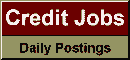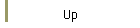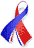DefaultRisk.com the web's biggest credit risk modeling resource.Export citation to:- HTML - Text (plain)- BibTeX - RIS - ReDIF

Correlation Expansions for CDO Pricing

by Paul Glasserman of Columbia University, and
Sira Suchintabandid of Columbia University

May 2007

Abstract: This paper develops numerical approximations for pricing collateralized debt obligations (CDOs) and other portfolio credit derivatives in the multifactor Normal Copula model. A key aspect of pricing portfolio credit derivatives is capturing dependence between the defaults of the elements of the portfolio. But, compared with an independent-obligor model, pricing in a model with correlated defaults is more challenging. Our approach strikes a balance by reducing the problem of pricing in a model with correlated defaults to calculations involving only independent defaults. We develop approximations based on power series expansions in a parameter that scales the underlying correlations. These expansions express a CDO tranche price in a multifactor model as a series of prices in independent-obligor models, which are easy to compute. The approach builds on a classical approximation for multivariate Gaussian probabilities; we introduce an alternative representation that greatly reduces the number of terms required to evaluate the coefficients in the expansion. We also apply this method to the underlying problem of computing joint probabilities of multivariate normal random variables for which the correlation matrix has a factor structure.

Keywords: CDO's, Copulas, Factor models, Multifactor, Default risk.

Published in: Journal of Banking & Finance, Vol. 31, No. 5, (May 2007), pp. 1375-1398.

Books Referenced in this paper:  (what is this?)